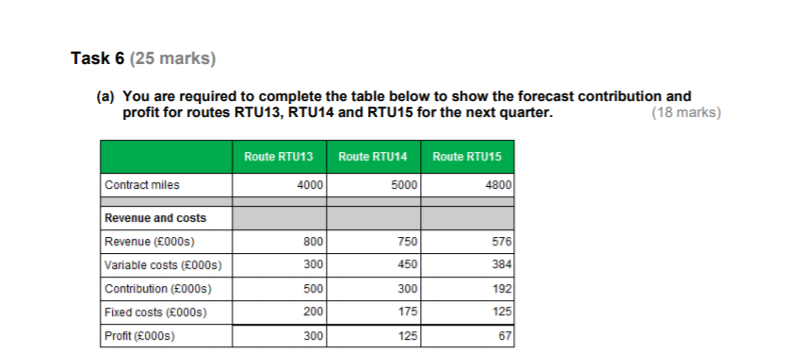# Exam question help

Hi would someone be able to explain to me how to get the following answers as my calculations are in the thousands not the hundreds?
My calculations are as follows:

Revenue=
4000 x 200 = 800000 but the answer says it should be 800
5000 x 150 (200-25%) = 750000 but the answer says it should be 750
4800 x 120 (200-40%)=576000 but the answer says it should be 576

Variable Costs=
4000 x 75 = 300000 but the answer says it should be 300
5000 x 90 (75+20%) = 45000 but the answer says it should be 450
4800 x 80 (75+5) = 384000 but the answer says it should be 384

Contribution=
Revenue – Variable costs
Fixed costs=
500,000 x 40%=200000 but the answer says it should be 200
500,000 x 35%=175000 but the answer says it should be 175
500,000 x 25%=125000 but the answer says it should be 125• The table states the answer is in £000s. This means when reading the answer you'd read it as thousands so 800 reads as 800,000 due to the units displayed. This can sometimes be used for bigger numbers as well such as £m for millions.
AAT Level 4, MAAT
ACCA in progress
F4- Passed Aug 2020
F5- Passed Dec 2020
F6- Passed Sep 2020
F7- Passed June 2021
F8 - Passed Sep 2021
F9 - Passed June 2021

SBL -
SBR - Passed Mar 22
ATX - Passed Dec 21
APM - Passed June 22
• Great thank you